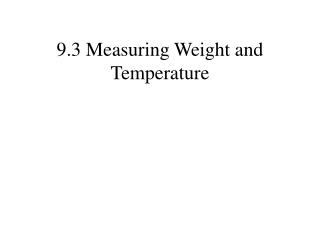DownloadDownload Presentation9.3 Measuring Weight and Temperature

# 9.3 Measuring Weight and Temperature

Télécharger la présentation## 9.3 Measuring Weight and Temperature

- - - - - - - - - - - - - - - - - - - - - - - - - - - E N D - - - - - - - - - - - - - - - - - - - - - - - - - - -
##### Presentation Transcript

1. 9.3 Measuring Weight and Temperature

2. Units of Weight in the English System 16 ounces (oz) = 1 pound (lb) 2000 pounds (lb) = 1 ton (T)

3. Common units for weight in the metric system This chart is found on page 519

4. One gram is the weight of 1 cubic centimeter of water. That is about the weight of a paperclip. One kilogram is about 2.2 pounds. kilogram hectogram dekagram gram decigram centigram milligram

5. To convert units within the metric system, multiply or divide by 10. Multiply by 10 as the units go to the right. Divide by 10 as the units go to the left. 0.1 kg =1 hg 0.1 g =1 dg 0.1 dg =1 cg 0.1 hg =1 dag 0.1 dag =1 g 0.1 cg =1 mg kg hg dag g dg cg mg 1 kg =10 hg 1 hg =10 dag 1 dag =10 g 1 g =10 dg 1 dg =10 cg 1 cg =10 mg

6. Convert 327.8 grams to kilograms Divide by 10 as the units go to the left. kg hg dag g dg cg mm

7. Convert 21.7 dekagrams to centigrams Multiply by 10 as the units go to the right. kg hg dag g dg cg mg

8. Convert 483.2 hectograms to milligrams Multiply by 10 as the units go to the right. kg hg dag g dg cg mg

9. Volume and Weight of Water in the Metric System Volume in Cubic Units Capacity Weight 1 cm3 = 1 mL 1 gram 1 dm3 = 1000 cm3 = 1 L 1 kilogram 1 m3 = 1 kL 1000 kilograms (1 tonne) What is the weight of the water in an aquarium that is 25 cm tall, 30 cm wide, and 60 cm long? The volume is 25 cm 𝗑 30 cm 60 cm = 45,000 cm3 The weight is 45,000 grams or 45 kilograms.

10. Weight Conversion between the English and Metric System 1 ounce (oz) ≈ 28 grams (g) 1 pound ≈ 0.45 kilogram (kg) 1 ton (T) ≈ 0.9 tonne (t) Convert 3.7 kilograms to pounds and ounces. 3.7 kilograms ≈ 8 pounds and 3.56 ounces.

11. Temperature Measurement In 1724 Daniel Fahrenheit (1686−1736) proposed a temperature scale. Zero was set as the temperature of ice being melted with a salt brine (he was probably making ice cream.) He chose 100 degrees to be the body temperature of a horse (horses have a more stable body temperature than humans.) 100° F = 0° F =

12. Temperature Measurement In 1742 Anders Celsius (1701−1744) proposed a temperature scale. Zero was set as the temperature of water boiling. He chose 100 degrees to be the temperature of water freezing. In 1744 after his death the scale was reversed. The Fahrenheit temperature of freezing water is 32°. The Fahrenheit temperature of boiling water is 212°. 100° C = 0° C =

13. Temperature Measurement To convert from Celsius to Fahrenheit, use the formula: To convert from Fahrenheit to Celsius, use the formula:

14. Temperature Measurement What is a normal human temperature, (98.6°F) in the Celsius scale? = 37°C

15. Temperature Measurement Convert −40°C to Fahrenheit. = −40°F

16. Temperature Measurement In 1848 William Thomson (Lord Kelvin) proposed a scale to measure heat. Zero Kelvin is the temperature where there is no heat. He used the same increments as Celsius. The temperature of zero Kelvin is the same as −273.15°C.